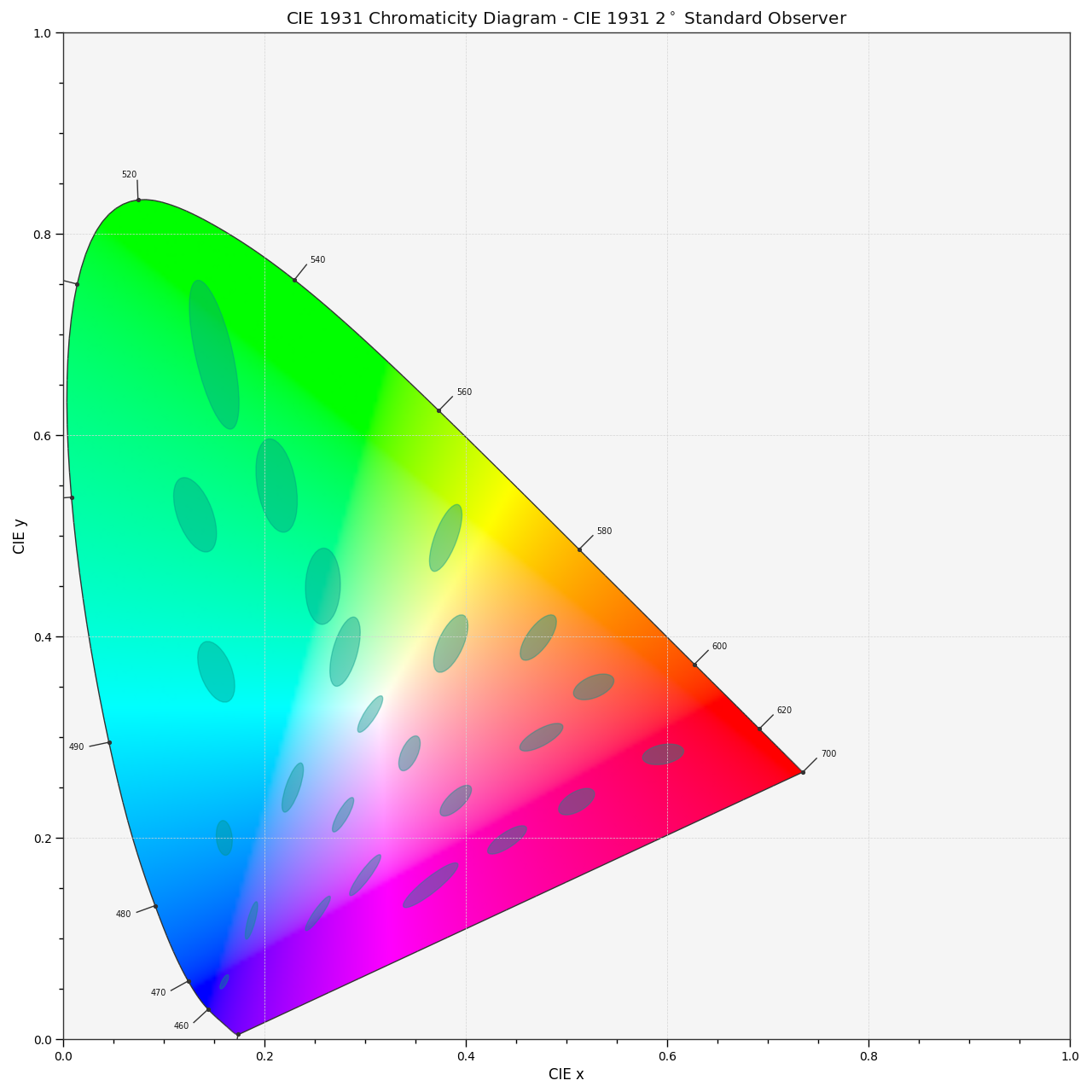colour.plotting.plot_ellipses_MacAdam1942_in_chromaticity_diagram_CIE1931(chromaticity_diagram_callable_CIE1931: Callable = plot_chromaticity_diagram_CIE1931, chromaticity_diagram_clipping: bool = False, ellipse_kwargs: dict | List[dict] | None = None, **kwargs: Any) [source]#

Plot MacAdam (1942) Ellipses (Observer PGN) in the CIE 1931 Chromaticity Diagram.

Parameters:
Returns:

Current figure and axes.

Return type:

`tuple`

Examples

```>>> plot_ellipses_MacAdam1942_in_chromaticity_diagram_CIE1931()
...
(<Figure size ... with 1 Axes>, <...Axes...>)
```